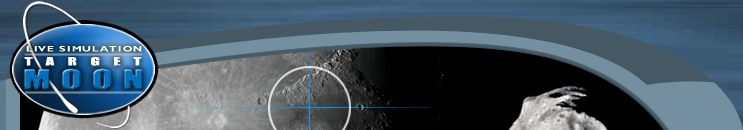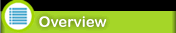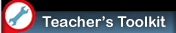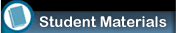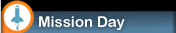OverviewStandards National Math Standards NM-NUM.: Understand numbers, ways of representing numbers, relationships among numbers, and number systems. Work flexibly with fractions, decimals, and percents to solve problems. NM-NUM.: Compute fluently and make reasonable estimates. Develop and analyze algorithms for computing with fractions, decimals, and integers and develop fluency in their use. NM-GEO.: Use visualization, spatial reasoning, and geometric modeling to solve problems. Recognize and apply geometric ideas and relationships in areas outside the mathematics classroom, such as art, science, and everyday life. NM-MEA.: Apply appropriate techniques, tools, and formulas to determine measurements. Select and apply techniques and tools to accurately find length, area, volume, and angle measures to appropriate levels of precision. Develop and use formulas to determine the circumference of circles and the area of triangles, parallelograms, trapezoids, and circles and develop strategies to find the area of more complex shapes. Solve simple problems involving rates and derived measurements for such attributes as velocity and density. NM-DATA.: Understand and apply basic concepts of probability. Use proportionality and a basic understanding of probability to make and test conjectures about the results of experiments and simulations. Download Microsoft Active Accessibility.Animation showing all of the innovative programs for digital learning that the Center for Educational Technologies has developed. Some of them include: EVA Alert, M.A.R.S., and Target Moon. The Center for Educational Technologies® at WJU has developed a series of innovative programs for digital learning. These programs provide teachers with an all-inclusive package comprised of curriculum aligned with state and national standards and a live “mission” via technology. The programs were designed around best practice models and principles derived from the latest educational research.